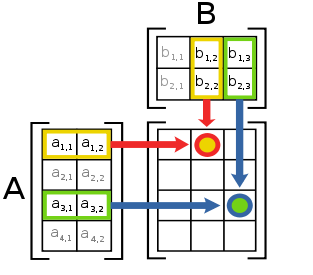# Glossary

## Matrix multiplication

When multiplying two matrices, $A$ and $B$, the elements of the rows in $A$ are multiplied with corresponding elements in columns in $B$. As a result, the number of elements in a row of $A$ should equal the number of elements in a column of $B$; by extension, the number of columns of $A$ should equal the number of rows of $B$.in the figure above, the resulting matrix $AB$ is computed one entry at a time. The values at the intersections marked with circles are:

$AB_{12}= (a_{11},a_{12})\cdot(b_{12},b_{22})=a_{11}b_{12} + a_{12}b_{22}\\ AB_{33}= (a_{31},a_{32})\cdot(b_{13},b_{23})=a_{31}b_{13} + a_{32}b_{23}$

As another example, here is the product of a $2 \times 2$ square matrix $A$ and a single column matrix (called a vector) $B$.

${A} = \begin{pmatrix} a & b \\ c & d \\ \end{pmatrix}, \quad {B} = \begin{pmatrix} x \\ y \\ \end{pmatrix}$

${AB} = \begin{pmatrix} a & b \\ c & d \\ \end{pmatrix} \begin{pmatrix} x \\ y \\ \end{pmatrix} = \begin{pmatrix} ax + by \\ cx + dy \\ \end{pmatrix}=\begin{pmatrix} ax + by \\ cx + dy \\ \end{pmatrix}$